# Buy and Sell Stock – IV | (DP – 38)

We are given an array Arr[] of length n. It represents the price of a stock on ‘n’ days. The following guidelines need to be followed:

1. We can buy and sell the stock any number of times.
2. In order to sell the stock, we need to first buy it on the same or any previous day.
3. We can’t buy a stock again after buying it once. In other words, we first buy a stock and then sell it. After selling we can buy and sell again. But we can’t sell before buying and can’t buy before selling any previously bought stock.
4. We can do at-most K transactions.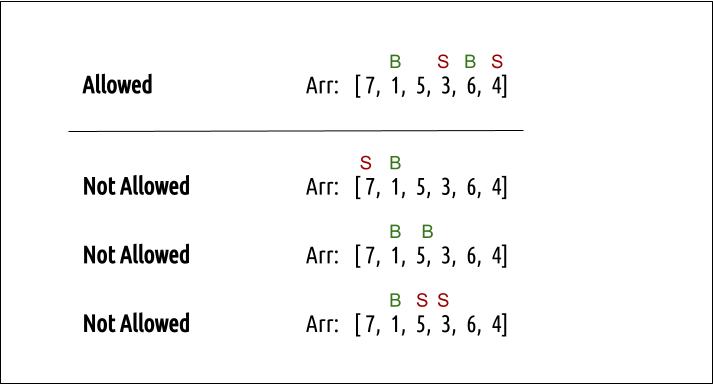Example: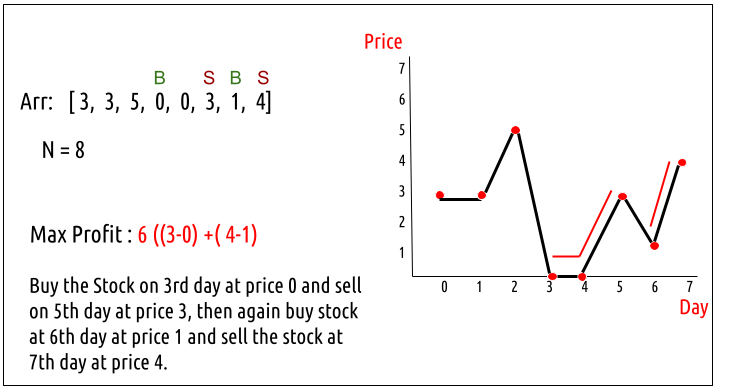K = 2

Disclaimer: Don’t jump directly to the solution, try it out yourself first.

Pre- Requisite: Buy and Sell Stock III

### Solution :

This question is quite similar to the question discussed in Buy and Sell Stock III. There we can do at most two transactions whereas here we can do at most K transactions. Therefore, we will modify that approach according to our needs in this section. Readers are highly advised to go through Buy and Sell Stock III first.

### Intuition:

Every day, we will have two choices, either to do nothing and move to the next day or to buy/sell (based on the last transaction and the number of transactions left) and find out the profit. Therefore we need to generate all the choices in order to compare the profit. As we need to try out all the possible choices, we will use recursion.

Steps to form the recursive solution:

We will first form the recursive solution by the three points mentioned in the Dynamic Programming Introduction

Step 1: Express the problem in terms of indexes.

We need to think in the terms of the number of days, therefore one variable will be the array index( say ind). Next, we need to respect the condition that we can’t buy a stock again, that is we need to first sell a stock, and then we can buy that again. Therefore we need a second variable ‘buy’ which tells us on a particular day whether we can buy or sell the stock. Next, we have a cap on the number of transactions that we can make. Here the initial cap is K.We need to always keep in mind this constraint. We can generalize the function as :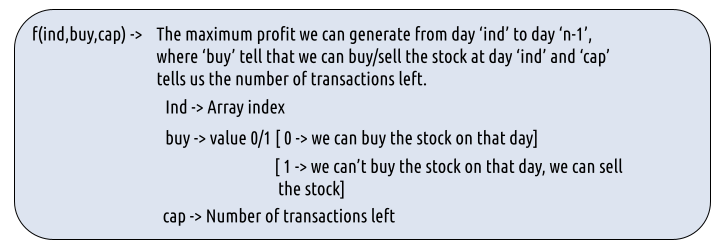Step 2: Try out all possible choices at a given index.

Every day, we have two choices:

• To either buy/sell the stock(based on the buy variable’s value and if ‘cap’ > 0).
• To do nothing and move on to the next day.

We need to generate all the choices. We will use the pick/non-pick technique as discussed in this video “Recursion on Subsequences”.

If we can buy the stock on a particular day, we have two options:

• Option 1: To do no transaction and move to the next day. In this case, the net profit earned will be 0 from the current transaction, and to calculate the maximum profit starting from the next day, we will recursively call f(ind+1,0, cap). As we have not bought the stock, the ‘buy’ variable value will still remain 0, indicating that we can buy the stock the next day. And the ‘cap’ variable will remain the same as if no transaction took place.
• Option 2: The other option is to buy the stock on the current day. In this case, the net profit earned from the current transaction will be -Arr[i]. As we are buying the stock, we are giving money out of our pocket, therefore the profit we earn is negative. To calculate the maximum profit starting from the next day, we will recursively call f(ind+1,1, cap). As we have bought the stock, the ‘buy’ variable value will change to 1, indicating that we can’t buy and only sell the stock the next day. As we have only bought the stock and not sold it the transaction remains incomplete and the ‘cap’ variable value remains unchanged.

Case 2: When buy == 1, we can sell the stock.

If we can buy the stock on a particular day, we have two options:

• Option 1: To do no transaction and move to the next day. In this case, the net profit earned will be 0 from the current transaction, and to calculate the maximum profit starting from the next day, we will recursively call f(ind+1,1, cap). As we have not bought the stock, the ‘buy’ variable value will still remain at 1, indicating that we can’t buy and only sell the stock the next day. And the ‘cap’ variable will remain the same as if no transaction took place.
• Option 2: The other option is to sell the stock on the current day. In this case, the net profit earned from the current transaction will be +Arr[i]. As we are selling the stock, we are putting the money into our pocket, therefore the profit we earn is positive. To calculate the maximum profit starting from the next day, we will recursively call f(ind+1,0,cap-1). As we have sold the stock, the ‘buy’ variable value will change to 0, indicating that we can buy the stock the next day. As we have sold the earlier bought stock, we make one complete transaction, therefore now we update the ‘cap’ variable’s value to cap-1.

Note: Buying and selling a stock together counts as one complete transaction.

The figure below gives us the summary: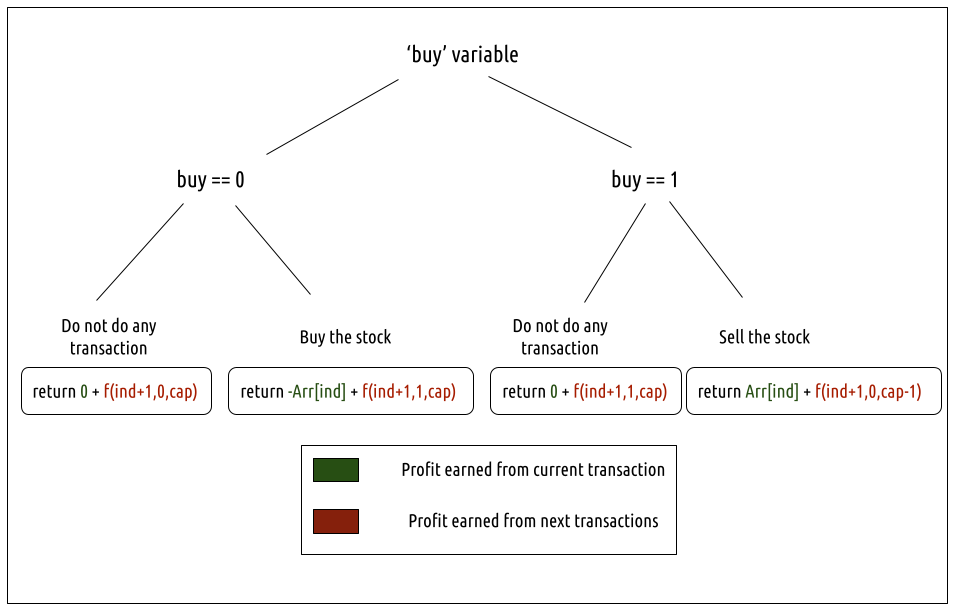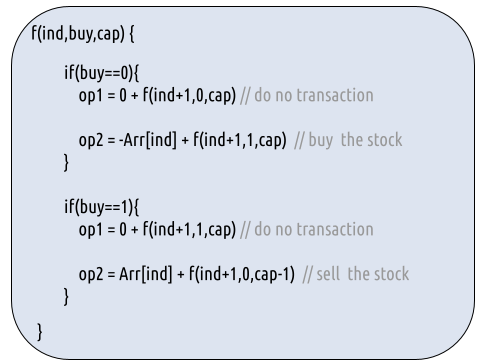Step 3:  Return the maximum

As we are looking to maximize the profit earned, we will return the maximum value in both cases.

Base Cases:

• If ind==n, it means we have finished trading on all days, and there is no more money that we can get, therefore we simply return 0.
• If cap==0, it means that we cannot make any more transactions. Therefore we return 0.

The final pseudocode after steps 1, 2, and 3: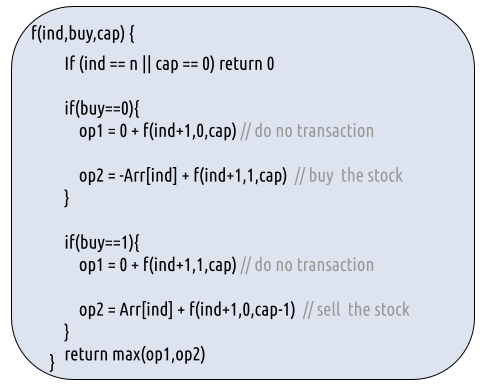Steps to memoize a recursive solution:

If we draw the recursion tree, we will see that there are overlapping subproblems. In order to convert a recursive solution the following steps will be taken:

1. Create a dp array of size [n]. The size of the input array is ‘n’, so the index will always lie between ‘0’ and ‘n-1’. The ‘buy’  variable can take only two values: 0 and 1 and the ‘cap’ variable can only take three variables 0, 1, and 2. Therefore we take the dp array as dp[n].
2. We initialize the dp array to -1.
3. Whenever we want to find the answer of particular parameters (say f(ind,buy,cap)), we first check whether the answer is already calculated using the dp array(i.e dp[ind][buy][cap]!= -1 ). If yes, simply return the value from the dp array.
4. If not, then we are finding the answer for the given value for the first time, we will use the recursive relation as usual but before returning from the function, we will set dp[ind][buy][cap] to the solution we get.

Code:

## C++ Code

``````#include <bits/stdc++.h>

using namespace std;

int getAns(vector<int>& Arr, int n, int ind, int buy, int cap, vector<vector<vector<int>>>& dp ){

if(ind==n || cap==0) return 0; //base case

int profit;

profit = max(0+getAns(Arr,n,ind+1,0,cap,dp),
-Arr[ind] + getAns(Arr,n,ind+1,1,cap,dp));
}

if(buy==1){// We can sell the stock
profit = max(0+getAns(Arr,n,ind+1,1,cap,dp),
Arr[ind] + getAns(Arr,n,ind+1,0,cap-1,dp));
}

}

int maximumProfit(vector<int>& prices, int n, int k)
{
// Creating a 3d - dp of size [n][k+1]
vector<vector<vector<int>>> dp(n,
vector<vector<int>>
(2,vector<int>(k+1,-1)));

return getAns(prices,n,0,0,k,dp);

}

int main() {

vector<int> prices = {3,3,5,0,0,3,1,4};
int n = prices.size();
int k = 2;

cout<<"The maximum profit that can be generated is "<<maximumProfit(prices, n,k);
}
``````

Output:

The maximum profit that can be generated is 6

Time Complexity: O(N*2*3)

Reason: There are N*2*K states therefore at max ‘N*2*K’ new problems will be solved.

Space Complexity: O(N*2*K) + O(N)

Reason: We are using a recursion stack space(O(N)) and a 3D array ( O(N*2*K)).

Steps to convert Recursive Solution to Tabulation one.

To convert the memoization approach to a tabulation one, create a dp array with the size [N+1][K+1].

Handling the base case:

Now, what the base condition in the recursive relation is:

if( ind == n || cap == 0) return 0

We handle this in the following way:

1. ind == n

When ind == n, the other two variables: cap and buy can take any value, therefore we can set the following two loops and set dp[n][buy][cap] = 0

1. cap == 0

When cap == 0, the other two variables: ind and cap can take any value, therefore we can set the following two loops and set dp[ind][buy] = 0.

Another hack is to initialize the entire 3D DP Array as 0. In this case, we need not worry about explicitly setting the base cases.

• First we declare the dp array of size [n+1][k+1] as zero.
• As we have initialized the array as 0, we have automatically set the base condition as explained above.
• Now, traverse the array in the opposite direction of that of the memoization technique. We will start from ind = n-1 -> ind =0.
• In every iteration copy the recursive code logic.
• At last dp ( maximum profit generated on ith day, when we can buy the stock on 0th day and can have a total 2 transactions) gives us the final answer.

Code:

## C++ Code

``````#include <bits/stdc++.h>

using namespace std;

int maximumProfit(vector<int>& Arr, int n, int k)
{
// Creating a 3d - dp of size [n+1][k+1] initialized to 0
vector<vector<vector<int>>> dp(n+1,
vector<vector<int>>
(2,vector<int>(k+1,0)));

// As dp array is initialized to 0, we have already covered the base case

for(int ind = n-1; ind>=0; ind--){
for(int cap=1; cap<=k; cap++){

-Arr[ind] + dp[ind+1][cap]);
}

if(buy==1){// We can sell the stock
Arr[ind] + dp[ind+1][cap-1]);
}
}
}
}

return dp[k];

}

int main() {

vector<int> prices = {3,3,5,0,0,3,1,4};
int n = prices.size();
int k =2;

cout<<"The maximum profit that can be generated is "<<maximumProfit(prices, n, k);
}
``````

Output:

The maximum profit that can be generated is 6

Time Complexity: O(N*2*k)

Reason: There are three nested loops that account for O(N*2*K) complexity.

Space Complexity: O(N*2*k)

Reason: We are using an external array of size ‘N*2*K’. Stack Space is eliminated.

Part 3: Space Optimization

If we closely look the relation,

We see that to calculate a value of a cell of the dp array, we need only the next row values(say ahead of ind+1). So, we don’t need to store an entire 2-D array. Hence we can space optimize it.

• We set a 2D vector ahead initialized to 0 (base condition) and another 2D
• Then we set three nested loops to calculate the cur array’s values.
• We replace dp[ind] with cur and dp[ind+1] with ahead in our tabulation code.
• After the inner loop execution, we set ahead as cur for the next outer loop iteration.

Code:

## C++ Code

``````#include <bits/stdc++.h>

using namespace std;

int maxProfit(vector<int>& Arr, int n, int k)
{

vector<vector<int>> cur(2,vector<int> (k+1,0));

for(int ind = n-1; ind>=0; ind--){
for(int cap=1; cap<=k; cap++){

}

if(buy==1){// We can sell the stock
}
}
}
}

}

int main() {

vector<int> prices = {3,3,5,0,0,3,1,4};
int n = prices.size();
int k=2;
cout<<"The maximum profit that can be generated is "<<maxProfit(prices, n,k);
}
``````

Output:

The maximum profit that can be generated is 6

Time Complexity: O(N*2*K)

Reason: There are three nested loops that account for O(N*2*K) complexity

Space Complexity: O(K)

Reason: We are using two external arrays of size ‘2*K’.